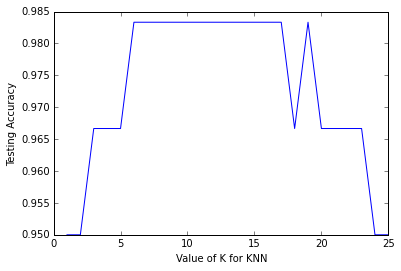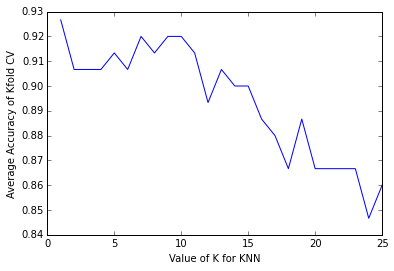## 内容概要¶

• 我们该怎样选择模型用于监督学习任务？
• 我们该如何选择调整得到最好的模型参数？
• 我们该如何对测试数据进行预测估计？

## 1. 使用整个数据集进行训练和测试¶

• 这里我们使用手中的整个数据集来训练模型
• 使用同样的数据集来测试模型，然后评估预测的结果和真实结果的差别
In :
from sklearn.datasets import load_iris

# create X(features) and y(response)
X = iris.data
y = iris.target


### Logistic regression¶

In :
from sklearn.linear_model import LogisticRegression
logreg = LogisticRegression()

logreg.fit(X, y)
y_pred = logreg.predict(X)
print "predicted response:\n",y_pred

predicted response:
[0 0 0 0 0 0 0 0 0 0 0 0 0 0 0 0 0 0 0 0 0 0 0 0 0 0 0 0 0 0 0 0 0 0 0 0 0
0 0 0 0 0 0 0 0 0 0 0 0 0 1 1 1 1 1 1 1 1 1 1 1 1 1 1 1 1 2 1 1 1 2 1 1 1
1 1 1 1 1 1 1 1 1 2 2 2 1 1 1 1 1 1 1 1 1 1 1 1 1 1 2 2 2 2 2 2 2 2 2 2 2
2 2 2 2 2 2 2 2 2 2 2 2 2 2 2 2 2 2 1 2 2 2 2 2 2 2 2 2 2 2 2 2 2 2 2 2 2
2 2]

In :
len(y_pred)

Out:
150

### 分类准确率¶

In :
from sklearn import metrics
print metrics.accuracy_score(y, y_pred)

0.96


### KNN(K=5)¶

In :
from sklearn.neighbors import KNeighborsClassifier
knn5 = KNeighborsClassifier(n_neighbors=5)
knn5.fit(X, y)
y_pred = knn5.predict(X)
print metrics.accuracy_score(y, y_pred)

0.966666666667


### KNN(K=1)¶

In :
knn1 = KNeighborsClassifier(n_neighbors=1)
knn1.fit(X, y)
y_pred = knn1.predict(X)
print metrics.accuracy_score(y, y_pred)

1.0


## 2. 分别设置训练集和测试集¶

• 将数据集分成两部分：训练集和测试集
• 使用训练集对模型进行训练
• 使用测试集进行模型的测试并评估性能如何
In :
print X.shape
print y.shape

(150, 4)
(150,)

In :
# 第一步：将X和y分割成训练和测试集
from sklearn.cross_validation import train_test_split
X_train, X_test, y_train, y_test = train_test_split(X, y, test_size=0.4, random_state=4)
# 这里的random_state参数根据给定的给定的整数，得到伪随机生成器的随机采样上面这个图告诉我们train_test_split函数的功能，将一个数据集分成两部分。这样使用不同的数据集对模型进行分别的训练和测试，得到的测试准确率能够更好的估计模型对于未知数据的预测效果。

In :
print X_train.shape
print X_test.shape

(90, 4)
(60, 4)

In :
print y_train.shape
print y_test.shape

(90,)
(60,)

In :
# 第二步：使用训练数据训练模型
logreg = LogisticRegression()
logreg.fit(X_train, y_train)

Out:
LogisticRegression(C=1.0, class_weight=None, dual=False, fit_intercept=True,
intercept_scaling=1, max_iter=100, multi_class='ovr',
penalty='l2', random_state=None, solver='liblinear', tol=0.0001,
verbose=0)
In :
# 第三步： 针对测试数据进行预测，并得到测试准确率
y_pred = logreg.predict(X_test)

print metrics.accuracy_score(y_test, y_pred)
# 可以尝试一下，对于上面不同数据集分割，得到的测试准确率不同

0.95


### 使用KNN算法¶

In :
# K=5

knn5 = KNeighborsClassifier(n_neighbors=5)
knn5.fit(X_train, y_train)
y_pred = knn5.predict(X_test)
print metrics.accuracy_score(y_test, y_pred)

0.966666666667

In :
# K=1

knn1 = KNeighborsClassifier(n_neighbors=1)
knn1.fit(X_train, y_train)
y_pred = knn1.predict(X_test)
print metrics.accuracy_score(y_test, y_pred)

0.95


In :
# 测试从K=1到K=25，记录测试准确率
k_range = range(1, 26)
test_accuracy = []

for k in k_range:
knn = KNeighborsClassifier(n_neighbors=k)
knn.fit(X_train, y_train)
y_pred = knn.predict(X_test)
test_accuracy.append(metrics.accuracy_score(y_test, y_pred))

In :
%matplotlib inline
import matplotlib.pyplot as plt

plt.plot(k_range, test_accuracy)
plt.xlabel("Value of K for KNN")
plt.ylabel("Testing Accuracy")

Out:
<matplotlib.text.Text at 0x71f1df0>### 模型参数的选择¶

In :
# 这里我们对未知数据进行预测
knn11 = KNeighborsClassifier(n_neighbors=11)
knn11.fit(X, y)

knn11.predict([3, 5, 4, 2])

Out:
array()

### 小结¶

• KNN的模型复杂度主要由K的值决定，K值越小，复杂度越高
• 训练准确度随着模型复杂度更加复杂而升高
• 测试准确率在模型过于复杂和过于简单的时候都比较低

## 3. K折交叉检验¶

K折将数据集分成K个部分，其中K-1组数据作为构建预测函数的训练之用，剩余的一组数据作为测试之用。

In :
from sklearn.cross_validation import KFold
import numpy as np

def cv_estimate(k, kfold=5):
cv = KFold(n = X.shape, n_folds=kfold)
clf = KNeighborsClassifier(n_neighbors=k)
score = 0
for train, test in cv:
clf.fit(X[train], y[train])
score += clf.score(X[test], y[test])
#print clf.score(X[test], y[test])
score /= kfold
return score

In :
# 测试从K=1到K=25，记录测试准确率
k_range = range(1, 26)
test_accuracy = []

for k in k_range:
test_accuracy.append(cv_estimate(k, 5))

In :
print test_accuracy

[0.92666666666666653, 0.90666666666666662, 0.90666666666666662, 0.90666666666666662, 0.91333333333333333, 0.90666666666666662, 0.92000000000000015, 0.91333333333333333, 0.92000000000000015, 0.92000000000000015, 0.91333333333333344, 0.89333333333333331, 0.90666666666666662, 0.90000000000000002, 0.90000000000000002, 0.88666666666666671, 0.88000000000000012, 0.86666666666666659, 0.88666666666666671, 0.86666666666666681, 0.86666666666666681, 0.86666666666666681, 0.86666666666666681, 0.84666666666666668, 0.85999999999999999]

In :
plt.plot(k_range, test_accuracy)
plt.xlabel("Value of K for KNN")
plt.ylabel("Average Accuracy of Kfold CV")

Out:
<matplotlib.text.Text at 0x7371530>